### Analysis of Maximum Likelihood Estimates

By default, PROC SURVEYLOGISTIC  displays the following information in the "Analysis of Maximum Likelihood Estimates" table:

• the degrees of freedom for Wald chi-square test

• maximum likelihood estimate of the parameter

• estimated standard error of the parameter estimate, computed as the square root of the corresponding diagonal element of the estimated covariance matrix

• Wald chi-square statistic, computed by squaring the ratio of the parameter estimate divided by its standard error estimate

•-value of the Wald chi-square statistic with respect to a chi-square distribution with one degree of freedom

• standardized estimate for the slope parameter, given by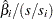, where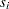is the total sample standard deviation for theth explanatory variable and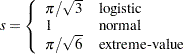You need to specify the STB option in the MODEL statement to obtain these estimates. Standardized estimates of the intercept parameters are set to missing.

• value of (e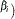for each slope parameter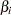if you specify the EXPB option in the MODEL statement. For continuous variables, this is equivalent to the estimated odds ratio for a one-unit change.

• label of the variable (if space permits) if you specify the PARMLABEL option in the MODEL statement. Due to constraints on the line size, the variable label might be suppressed in order to display the table in one panel. Use the SAS system option LINESIZE= to specify a larger line size to accommodate variable labels. A shorter line size can break the table into two panels, allowing labels to be displayed.